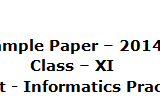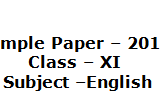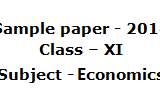## Sample Paper of Information Practice 2014 for class 11, CBSE. Paper No.3Sample Paper – 2014 Class – XI Subject – Informatics Practices Q1.Write  key feature of MySql (Any Four)                                                                                          2 Q2.Write Classification of Sql Statements                                                                                              2 Q3. Write any four important data type with example                                                                    2 Q4. Write uses of comment in MySql with an example                                                                   2 Q5Describe Following Comamnd with proper example                                                                   4 Desc Table  Distinct Where clause Create Table Q6.What is Relation Operator .Describe with example                                                                    2 Q7. What...

## Sample Paper of Information Practice 2014 for class 11, CBSE. Paper No.2Sample paper – 2014 Class – XI Subject – Informatics Practices         Max. Marks : 70                                                                                   Time            : 3 Hrs.     Note :  Answer all the questions. SECTION – A ( 20 Marks ) Q.1 Answer the following questions :                                                                                         ( 5 x 2 = 10 ) (a) What are the Strengths and Weakness of a Computer. (b) What are Input Devices ? Explain any three. (c) Explain...

## Sample Paper of Information Practice 2014 for class 11, CBSE. Paper No.2Sample Paper – 2014 Class – XI Subject – INFORMATION PRACTICES Time Allowed: 3 hours                                                                                          Maximum Marks: 70   Note: This question paper is divided into 3 sections. Section A consists of 30 marks. Section B and Section C are of 20 marks each. Answer the questions after carefully reading the text. Give example wherever required.  SECTION – A         1. Answer the following questions:   (a) What are the...

## Sample Paper of Information Practice 2014 for class 11, CBSE. Paper No.1Sample Paper – 2014 Class – XI Subject – Informatics Practices Time: 3 Hrs                                                                                                                         M.M: 70 Q1. (I) what will be the result of the following expressions?                                     4 ++i<=10 i++<=10 Y=++x Y=x++ Assume value of i = 10 and x= 42 (II) What output the following code fragment produce?                                     6  int val , res, n=1000; res=n+Val>1750? 400:200; system.out.println(res);   If the input is 2000 If the input is 1000 If the...

## Sample Paper of English 2014 for class 11, CBSE. Paper No.1Sample Paper – 2014 Class – XI Subject –English   Read the following passage carefully and answer the questions by writing The options that you consider the most appropriate in your answer sheet. Fifty years ago people ate ice cream only in summer .Now it is eaten all the year round. It originated in the Orient, centuries before English schoolboys first tasted it. Marco Polo saw people eating ice cream there...

## Sample Paper of Economics 2014 for class 11, CBSE. Paper No.3Sample Paper – 2014 Class – XI Subject – Economics     Time allowed: 3 hours                                                                                   Maximum Marks: 100   General instructions: (i)    All questions in both the sections are compulsory, (ii)   Marks for questions are indicated against each. (iii)  Questions carrying 1 mark for each part are required to be answered in one sentence each. (iv)  Questions carrying 3 marks are required to be answered not exceed 60...